## What are Moving Averages

Moving averages are one of the most used tool in financial (equities, forex, commodities) and can be used also to trade binary options.

Moving averages are be used to:

• identify the main trend of the underlying asset price
• pinpoint entry and exit points
• design dynamic support and resistance price levels.

The two most popular types of moving averages are the Simple Moving Average (SMA) and the Exponential Moving Average (EMA).

Graphically, they look like the below chart.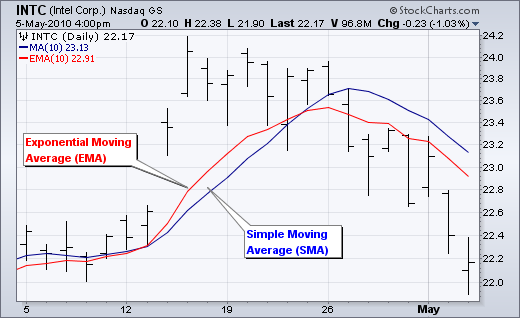## The Lag Factor

The longer the moving average, the higher the lag between the moving average and the price

A 10-day exponential moving average will follow prices quite closely and turn shortly after prices turn than a 30-day moving average.

A 100-day moving average is quite late in following the price turns, as it is calculated over a much longer time span and with a much older price data, going back 100 days.

The chart below shows 10-day, 50-day and 100-day moving averages.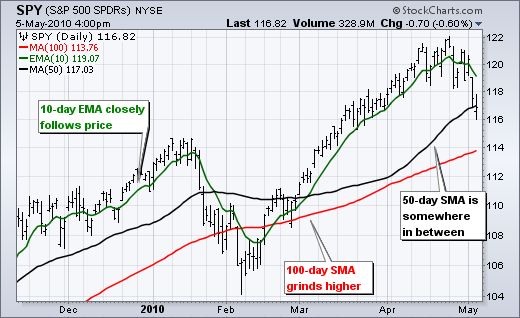## Simple versus Exponential Moving Averages

### Simple Moving Average Calculation

A simple moving average is formed by computing the average closing price of a security over a specific number of periods.

A 10-day simple moving average is the sum of closing prices of the last 10 days divided by 10.

Every day, the oldest day closing price dropped from the calcualtion, and the most recent closing price is added.

Each closing price has the the same weight, meaning that in a 10-period moving average the price of day 1 is as important as the price of day 10, even if older.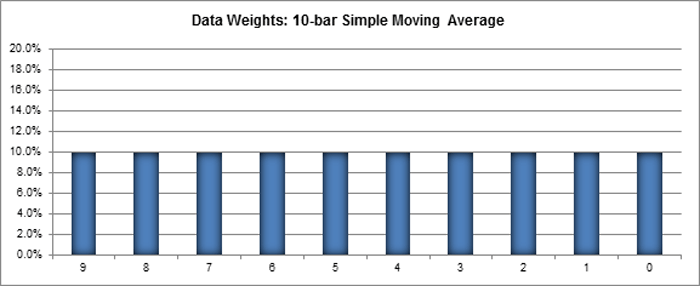### Exponential Moving Average Calculation

Exponential moving averages reduce the lag by applying more weight to recent prices and less to the oldest prices, as indicated by the chart below referring to the 10-day exponential moving average.

The 10-period exponential moving average applies an 18.18% weighting to the most recent price.

A 20-period EMA applies a 9.52% weighing to the most recent price (2/(20+1) = .0952).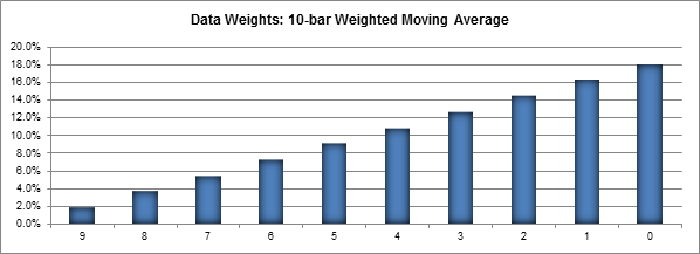### Even though there are clear differences between simple moving averages and exponential moving averages, one is not necessarily better than the other.

Exponential moving averages have less lag and are therefore more sensitive to recent prices.

Exponential moving averages will turn before simple moving averages.

Simple moving averages, on the other hand, represent a true average of prices for the entire time period.

As such, simple moving averages may be better suited to identify support or resistance levels.

Moving average preference depends on objectives, analytical style and time horizon.

Chartists should experiment with both types of moving averages as well as different timeframes to find the best fit.

The chart below shows IBM with the 50-day SMA in red and the 50-day EMA in green.

Both peaked in late January, but the decline in the EMA was sharper than the decline in the SMA.

The EMA turned up in mid February, but the SMA continued lower until the end of March.

Notice that the SMA turned up over a month after the EMA.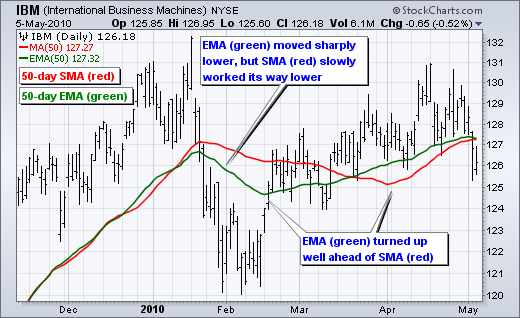### Lengths and Timeframes

The length of the moving average is the most important setting for a trader, because it determines the quality and reliability of the trading signals generated.

Short moving averages (5-20 periods) are best suited for short-term trading.

Chartists interested in medium-term trends would opt for longer moving averages that might extend 20-60 periods.

Long-term investors will prefer moving averages with 100 or more periods.

The 200-day moving average is perhaps the most popular.

Because of its length, this is clearly a long-term moving average.

Next, the 50-day moving average is quite popular for the medium-term trend.

Many chartists use the 50-day and 200-day moving averages together.

Short-term, a 10-day moving average was quite popular in the past because it was easy to calculate.

Salva

### A study made by ETF HQ shows that:

"As a general rule we can conclude that the Exponential Moving Average is superior to both the Simple Moving Average and the Double Exponential Moving Average"

and

"the 75 Day Exponential Moving Average with End of Week Signals as the ultimate winner because it also produced good returns on the short side of the market"

and

"the 25 Day Exponential Moving Average with End of Day Signals as the ultimate winner for Short trades because it produced the best returns out of the finalists"

Probably the best way is to test these findings and make your own analysis.

A relatively low cost way to make your own research is Expert Advisor Builder for Metatrader and Tradestation.### Trend Identification

The direction of the moving average conveys important information about prices.

The chart below reported is self-explanatory.

Example of moving averages as indicators of trend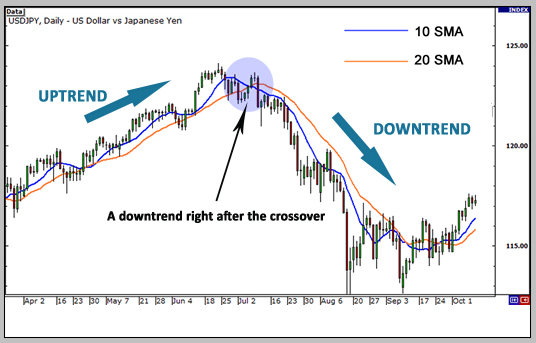Salva

## Price Crossovers

One of the easiest way a moving averages can be used to generate signals is through the very simple crossover between the price and the moving average.

• If the price moves above the moving average: bullish signal
• If the price moves below the moving average: bearish signal

Examples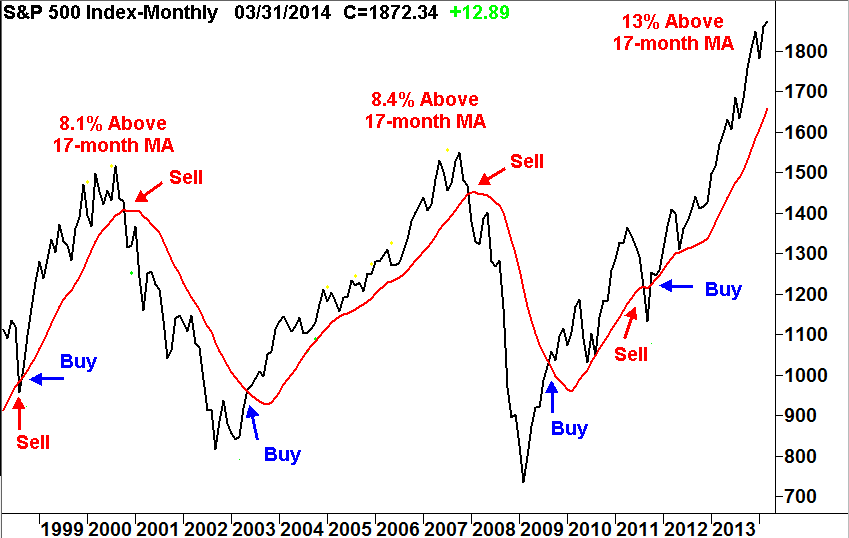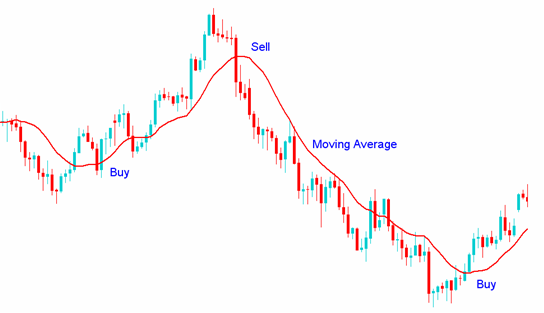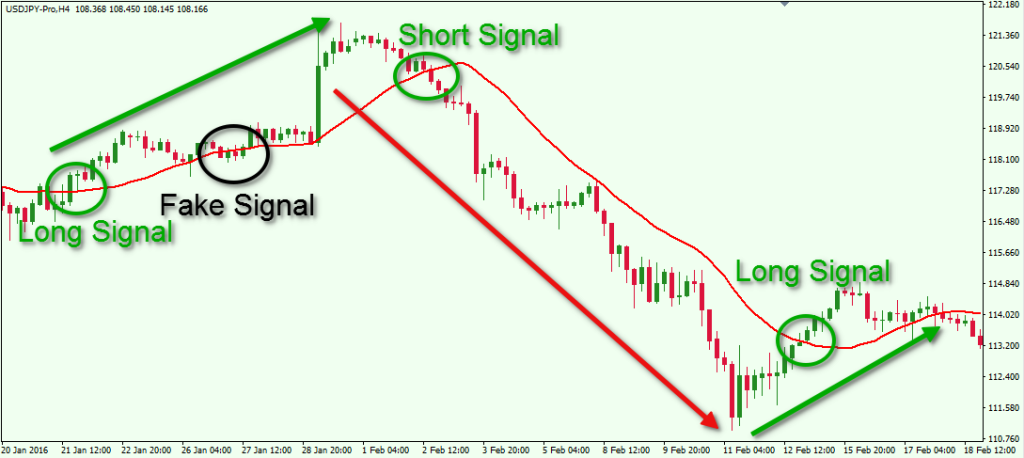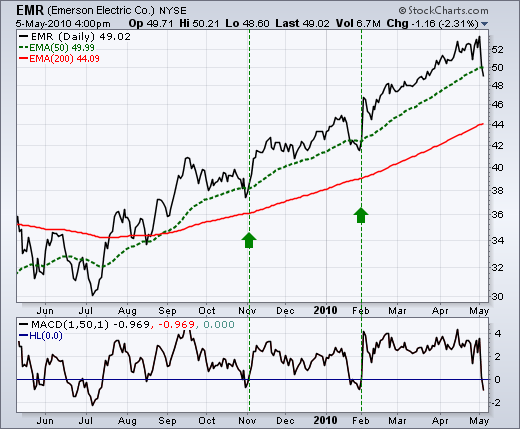### How to use moving averages: Double Crossover

Another way to use moving averages to generate signals is through the crossover between two moving averages.

This is called “double crossover method”.

One moving average is relatively short and the other relatively long.

As with all moving averages, the general length of the moving average defines the timeframe for the system.

Examples of double crossovers:

A system using a 5-day EMA and 35-day EMA is considered for short-term trading.

A system using a 50-day SMA and 200-day SMA is considered medium-term, and even long-term.

Golden cross

This is a bullish signal: the shorter moving average crosses above the longer moving average; this is also known as a golden cross.

Dead cross

Bearish signal: the shorter moving average crosses below the longer moving average; this is known as a dead cross.

The only thing about the double crossover is that it produces relatively late signals.

The longer the moving average periods, the greater the lag in the signals.

These signals work great when a good trend takes hold.

A moving average crossover system will produce lots of whipsaws in the absence of a strong trend.

Examples of double crossovers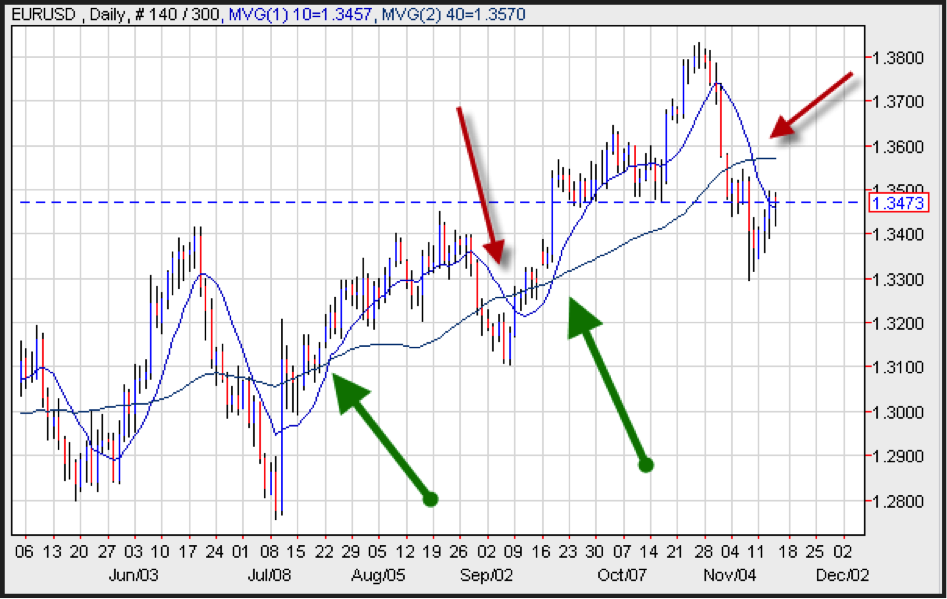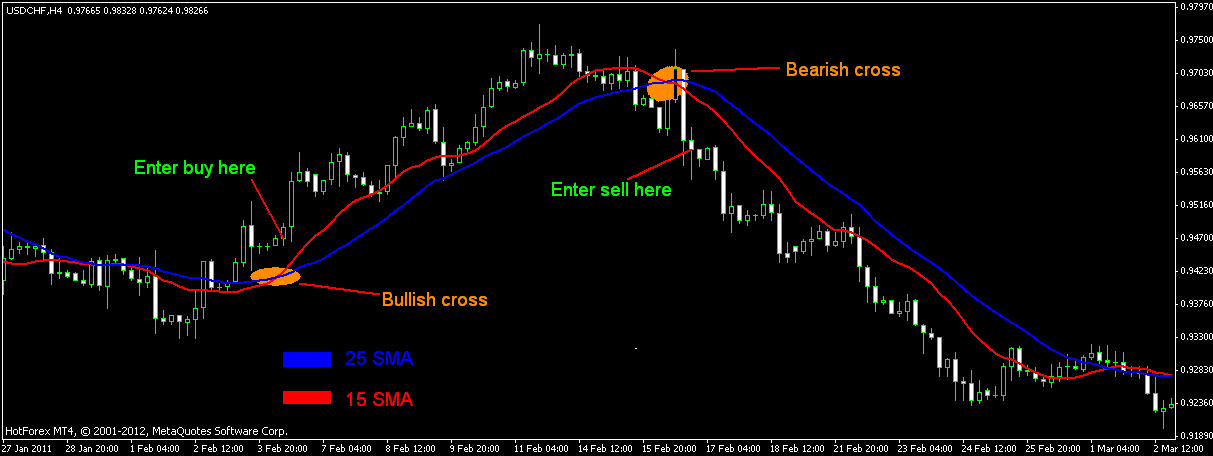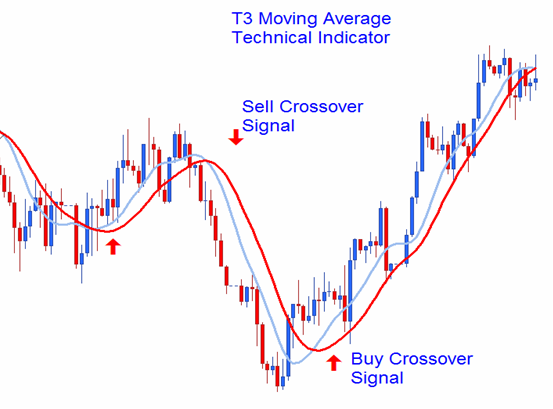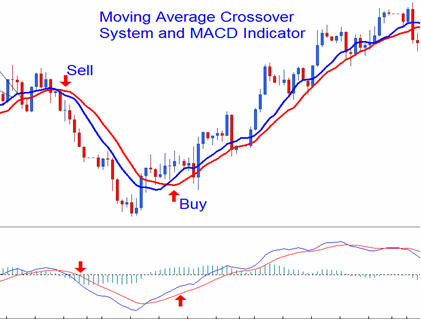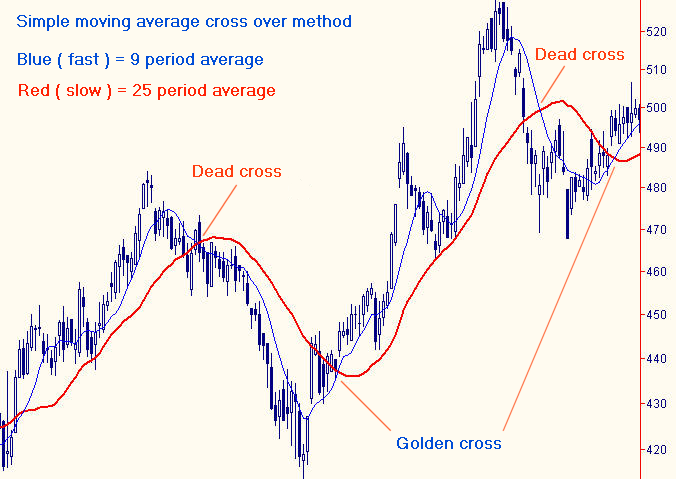### Best double cross = EMA 13 with EMA 48,5

A recent study by ETF HQ has proven that under specific assumptions the highest returns have been produced by the golden cross between the 13 and 48,5 EMA.

### Conclusions

Due to their own nature, moving averages follow the trend, they are one step behind the price movement.

On the other side, they probably are the best tool to trade in the direction of the trend.

Once in a trend, moving averages will give you a lot of trading satisfactions; in sideways market though, they can generate false entry and exit signals.

### Choose Speedlines trading system to pick the best entry points

Even if the MACD is a better tool to determine the entry point than the Bollinger Bands and the RSI, you need a trading system that tells you exactly when to enter:

Buy Speedlines, The Binary Optioner's flagship trading system.